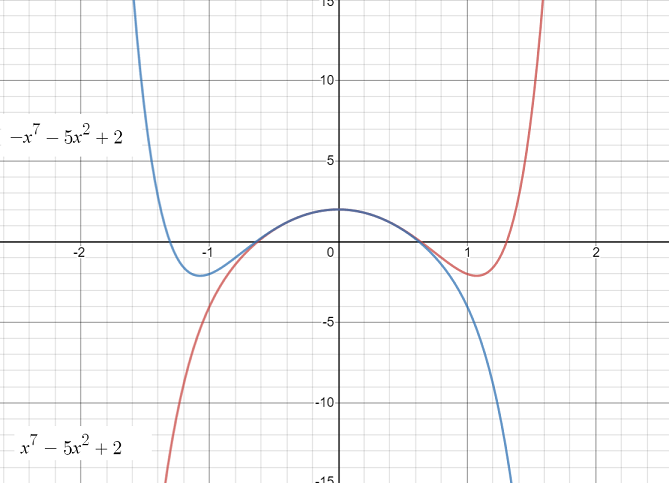# Septic Function (7th Degree Polynomial)

A septic function (also called a 7th degree polynomial) is a polynomial function with a degree of 7 (a “degree” is just the number of the highest exponent). All of the following are septic functions:

1. x7 – 3x6 – 7x4 + 21x3 – 8x + 24
2. x7 + 10x4 – 7x
3. x7 + x2

## More septic function examples: Monomial and Trinomial

Polynomials are named by degree and number of terms. A trinomial has 3 terms, a binomial has two terms and a monomial has one term. All of the following are septic functions:

1. 7th degree trinomial function: x7 + 2x4 + x
2. 7th degree binomial function: x7 + 4x2
3. 7th degree monomial function: x7

## Graph of a Septic FunctionGraph of a septic function with 7 roots (x-axis crossings) and 6 critical points (local maximums and local minimums). Image: Melikamp | Wikimedia Commons
End behavior: If a sepctic function has a negative leading coefficient (the “leading coefficient” is the first one), the function will start from the top (i.e. it will travel down) and end at the bottom (also continuing to travel down). The opposite is true for functions with positive leading coefficients: the graph travels upwards at both the beginning and end.The blue graph (negative leading coefficient) travels down at the beginning and end; A positive leading coefficient will result in a graph that travels up at the beginning and end (red graph).

## Derivative of a Septic Function

The derivative of a septic function is a sextic function (i.e. a polynomial function with 6 degrees.

Example: Find the derivative of f(x) = x7 – 3x6 – 7x4 + 21x3 – 8x + 24

Solution:
f′(x) = x7 – 3x6 – 7x4 + 21x3 – 8x + 24
= 7x6 – 18x5 – 28x2 + 63x2 – 8

Note: If you aren’t sure how I found the derivative, see: The Power rule: How to find derivatives for exponents.

## References

Image 1: Melikamp [CC BY-SA 3.0 (https://creativecommons.org/licenses/by-sa/3.0)]
Image 2: Desmos.com

CITE THIS AS:
Stephanie Glen. "Septic Function (7th Degree Polynomial)" From StatisticsHowTo.com: Elementary Statistics for the rest of us! https://www.statisticshowto.com/septic-function-7th-degree-polynomial/